College Algebra with Corequisite Support 2e

# 5.6Rational Functions

### Learning Objectives

In this section, you will:

• Use arrow notation.
• Solve applied problems involving rational functions.
• Find the domains of rational functions.
• Identify vertical asymptotes.
• Identify horizontal asymptotes.
• Graph rational functions.

### Corequisite Skills

#### Learning Objectives

• Determine the values for which a rational expression is undefined (IA 7.1.1)
• Find x- and y-intercepts (IA 3.1.4)

### Rational Expression

A rational expression is an expression of the form $pq,pq,$ where p and q are polynomials and $q≠0.q≠0.$

Here are some examples of rational expressions:

$−24565x12y4x+1x2−94x2+3x−12x−8 −24565x12y4x+1x2−94x2+3x−12x−8$
##### Practice Makes Perfect

Evaluate the following expression for the given values $5x-10x+35x-10x+3$

1.

$x=3x=3$

2.

$x=2x=2$

3.

$x=-3x=-3$

4.

Why do we have a problem with evaluating this expression for $x=−3x=−3$ but not for $x=2x=2$ ?

We say that this rational expression is undefined because its denominator equals 0.

### How To

Determine the values for which a rational expression is undefined.

1. Step 1. Set the denominator equal to zero.
2. Step 2. Solve the equation.

### Example 1

Determine the value for which the rational expression $x+32x2+9x-5x+32x2+9x-5$ is undefined

##### Practice Makes Perfect

Determine the value for which each rational expression is undefined.

5.

$3y25x3y25x$

6.

$3x+52x-33x+52x-3$

7.

$m-5m2+m-6m-5m2+m-6$

8.

$2aa2-92aa2-9$

9.

Determine the value for which the function $f(x)=3x-2f(x)=3x-2$ is undefined.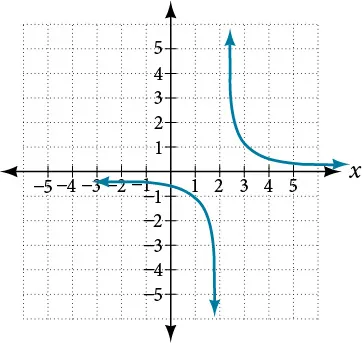What is the connection between the value you found and the graph of this function?

Because function is not defined at $x=2x=2$ , we say that the Domain of this function is

The domain of a rational function includes all real numbers except those that cause the denominator to equal zero.

10.

Determine the domain of the function and express using interval notation.

1. $f(x)=x3x-1f(x)=x3x-1$
2. $f(x)=3x2-5x+6f(x)=3x2-5x+6$

### Vocabulary

The $xx$-intercept is a point where the graph intersects the ________ axis. The $yy$-coordinate at this point is always ________.

The $yy$-intercept is a point where the graph intersects the ________ axis. The $xx$-coordinate at this point is always ________.

### How To

Find the x-intercept and y-intercept of a line.

Example: $3x−4y=93x−4y=9$

Find the $xx$ -intercept and $yy$ -intercept of a line.

 To find the $xx$ -intercept, replace $yy$ with zero and find $xx$ $xx$ -int: $3x−4(0)=93x−4(0)=9$ , $3x=93x=9$ , $x=3x=3$ (3, 0) To find the $yy$ -intercept, replace $xx$ with zero and find $yy$ $yy$ -int: $3(0)–4y=93(0)–4y=9$ , $–4y=9–4y=9$ , $y=–94y=–94$ (0, $–94–94$ )
##### Practice Makes Perfect

Find $xx$ - and $yy$ -intercept of each of the following functions. Express each as an ordered pair.

11.

12.

$y=5x-1y=5x-1$

13.

$y=1(x-2)+3y=1(x-2)+3$

Suppose we know that the cost of making a product is dependent on the number of items, $x, x,$ produced. This is given by the equation $C(x)=15,000x−0.1 x 2 +1000. C(x)=15,000x−0.1 x 2 +1000.$ If we want to know the average cost for producing $x x$ items, we would divide the cost function by the number of items, $x. x.$

The average cost function, which yields the average cost per item for $x x$ items produced, is

$f(x)= 15,000x−0.1 x 2 +1000 x f(x)= 15,000x−0.1 x 2 +1000 x$

Many other application problems require finding an average value in a similar way, giving us variables in the denominator. Written without a variable in the denominator, this function will contain a negative integer power.

In the last few sections, we have worked with polynomial functions, which are functions with non-negative integers for exponents. In this section, we explore rational functions, which have variables in the denominator.

### Using Arrow Notation

We have seen the graphs of the basic reciprocal function and the squared reciprocal function from our study of toolkit functions. Examine these graphs, as shown in Figure 1, and notice some of their features.

Figure 1

Several things are apparent if we examine the graph of $f(x)= 1 x . f(x)= 1 x .$

1. On the left branch of the graph, the curve approaches the x-axis
2. As the graph approaches $x=0 x=0$ from the left, the curve drops, but as we approach zero from the right, the curve rises.
3. Finally, on the right branch of the graph, the curves approaches the x-axis

To summarize, we use arrow notation to show that $x x$ or $f(x) f(x)$ is approaching a particular value. See Table 1.

Symbol Meaning
$x→ a − x→ a −$ $x x$ approaches $a a$ from the left ( $x but close to $a a$ )
$x→ a + x→ a +$ $x x$ approaches $a a$ from the right ( $x>a x>a$ but close to $a a$ )
$x→∞ x→∞$ $x x$ approaches infinity ( $x x$ increases without bound)
$x→−∞ x→−∞$ $x x$ approaches negative infinity ( $x x$ decreases without bound)
$f(x)→∞ f(x)→∞$ the output approaches infinity (the output increases without bound)
$f(x)→−∞ f(x)→−∞$ the output approaches negative infinity (the output decreases without bound)
$f(x)→a f(x)→a$ the output approaches $a a$
Table 1

#### Local Behavior of $f(x)= 1 x f(x)= 1 x$

Let’s begin by looking at the reciprocal function, $f(x)= 1 x . f(x)= 1 x .$ We cannot divide by zero, which means the function is undefined at $x=0; x=0;$ so zero is not in the domain. As the input values approach zero from the left side (becoming very small, negative values), the function values decrease without bound (in other words, they approach negative infinity). We can see this behavior in Table 2.

 $x x$ –0.1 –0.01 –0.001 –0.0001 $f(x)= 1 x f(x)= 1 x$ –10 –100 –1000 –10,000
Table 2

We write in arrow notation

As the input values approach zero from the right side (becoming very small, positive values), the function values increase without bound (approaching infinity). We can see this behavior in Table 3.

 $x x$ 0.1 0.01 0.001 0.0001 $f(x)= 1 x f(x)= 1 x$ 10 100 1000 10,000
Table 3

We write in arrow notation

See Figure 2.

Figure 2

This behavior creates a vertical asymptote, which is a vertical line that the graph approaches but never crosses. In this case, the graph is approaching the vertical line $x=0 x=0$ as the input becomes close to zero. See Figure 3.

Figure 3

### Vertical Asymptote

A vertical asymptote of a graph is a vertical line $x=a x=a$ where the graph tends toward positive or negative infinity as the input approaches $a a$ from either the left or the right. We write

#### End Behavior of $f(x)= 1 x f(x)= 1 x$

As the values of $x x$ approach infinity, the function values approach 0. As the values of $x x$ approach negative infinity, the function values approach 0. See Figure 4. Symbolically, using arrow notation

Figure 4

Based on this overall behavior and the graph, we can see that the function approaches 0 but never actually reaches 0; it seems to level off as the inputs become large. This behavior creates a horizontal asymptote, a horizontal line that the graph approaches as the input increases or decreases without bound. In this case, the graph is approaching the horizontal line $y=0. y=0.$ See Figure 5.

Figure 5

### Horizontal Asymptote

A horizontal asymptote of a graph is a horizontal line $y=b y=b$ where the graph approaches the line as the inputs increase or decrease without bound. We write

### Example 1

#### Using Arrow Notation

Use arrow notation to describe the end behavior and local behavior of the function graphed in Figure 6.

Figure 6

### Try It #1

Use arrow notation to describe the end behavior and local behavior for the reciprocal squared function.

### Example 2

#### Using Transformations to Graph a Rational Function

Sketch a graph of the reciprocal function shifted two units to the left and up three units. Identify the horizontal and vertical asymptotes of the graph, if any.

#### Analysis

Notice that horizontal and vertical asymptotes are shifted left 2 and up 3 along with the function.

### Try It #2

Sketch the graph, and find the horizontal and vertical asymptotes of the reciprocal squared function that has been shifted right 3 units and down 4 units.

### Solving Applied Problems Involving Rational Functions

In Example 2, we shifted a toolkit function in a way that resulted in the function $f(x)= 3x+7 x+2 . f(x)= 3x+7 x+2 .$ This is an example of a rational function. A rational function is a function that can be written as the quotient of two polynomial functions. Many real-world problems require us to find the ratio of two polynomial functions. Problems involving rates and concentrations often involve rational functions.

### Rational Function

A rational function is a function that can be written as the quotient of two polynomial functions

$f(x)= P(x) Q(x) = a p x p + a p−1 x p−1 +...+ a 1 x+ a 0 b q x q + b q−1 x q−1 +...+ b 1 x+ b 0 ,Q(x)≠0 f(x)= P(x) Q(x) = a p x p + a p−1 x p−1 +...+ a 1 x+ a 0 b q x q + b q−1 x q−1 +...+ b 1 x+ b 0 ,Q(x)≠0$

### Example 3

#### Solving an Applied Problem Involving a Rational Function

After running out of pre-packaged supplies, a nurse in a refugee camp is preparing an intravenous sugar solution for patients in the camp hospital. A large mixing tank currently contains 100 gallons of distilled water into which 5 pounds of sugar have been mixed. A tap will open pouring 10 gallons per minute of distilled water into the tank at the same time sugar is poured into the tank at a rate of 1 pound per minute. Find the ratio of sugar to water, in pounds per gallon in the tank after 12 minutes. Is that a greater ratio of sugar to water, in pounds per gallon than at the beginning?

### Try It #3

There are 1,200 first-year and 1,500 second-year students at a rally at noon. After 12 p.m., 20 first-year students arrive at the rally every five minutes while 15 second-year students leave the rally. Find the ratio of first-year to second-year students at 1 p.m.

### Finding the Domains of Rational Functions

A vertical asymptote represents a value at which a rational function is undefined, so that value is not in the domain of the function. A reciprocal function cannot have values in its domain that cause the denominator to equal zero. In general, to find the domain of a rational function, we need to determine which inputs would cause division by zero.

### Domain of a Rational Function

The domain of a rational function includes all real numbers except those that cause the denominator to equal zero.

### How To

Given a rational function, find the domain.

1. Set the denominator equal to zero.
2. Solve to find the x-values that cause the denominator to equal zero.
3. The domain is all real numbers except those found in Step 2.

### Example 4

#### Finding the Domain of a Rational Function

Find the domain of $f(x)= x+3 x 2 −9 . f(x)= x+3 x 2 −9 .$

#### Analysis

A graph of this function, as shown in Figure 8, confirms that the function is not defined when $x=±3. x=±3.$

Figure 8

There is a vertical asymptote at $x=3 x=3$ and a hole in the graph at $x=−3. x=−3.$ We will discuss these types of holes in greater detail later in this section.

### Try It #4

Find the domain of $f(x)= 4x 5(x−1)(x−5) . f(x)= 4x 5(x−1)(x−5) .$

### Identifying Vertical Asymptotes of Rational Functions

By looking at the graph of a rational function, we can investigate its local behavior and easily see whether there are asymptotes. We may even be able to approximate their location. Even without the graph, however, we can still determine whether a given rational function has any asymptotes, and calculate their location.

#### Vertical Asymptotes

The vertical asymptotes of a rational function may be found by examining the factors of the denominator that are not common to the factors in the numerator. Vertical asymptotes occur at the zeros of such factors.

### How To

Given a rational function, identify any vertical asymptotes of its graph.

1. Factor the numerator and denominator.
2. Note any restrictions in the domain of the function.
3. Reduce the expression by canceling common factors in the numerator and the denominator.
4. Note any values that cause the denominator to be zero in this simplified version. These are where the vertical asymptotes occur.
5. Note any restrictions in the domain where asymptotes do not occur. These are removable discontinuities, or “holes.”

### Example 5

#### Identifying Vertical Asymptotes

Find the vertical asymptotes of the graph of $k(x)= 5+2 x 2 2−x− x 2 . k(x)= 5+2 x 2 2−x− x 2 .$

#### Removable Discontinuities

Occasionally, a graph will contain a hole: a single point where the graph is not defined, indicated by an open circle. We call such a hole a removable discontinuity.

For example, the function $f(x)= x 2 −1 x 2 −2x−3 f(x)= x 2 −1 x 2 −2x−3$ may be re-written by factoring the numerator and the denominator.

$f(x)= ( x+1 )( x−1 ) ( x+1 )( x−3 ) f(x)= ( x+1 )( x−1 ) ( x+1 )( x−3 )$

Notice that $x+1 x+1$ is a common factor to the numerator and the denominator. The zero of this factor, $x=−1, x=−1,$ is the location of the removable discontinuity. Notice also that $x–3 x–3$ is not a factor in both the numerator and denominator. The zero of this factor, $x=3, x=3,$ is the vertical asymptote. See Figure 10. [Note that removable discontinuities may not be visible when we use a graphing calculator, depending upon the window selected.]

Figure 10

### Removable Discontinuities of Rational Functions

A removable discontinuity occurs in the graph of a rational function at $x=a x=a$ if $a a$ is a zero for a factor in the denominator that is common with a factor in the numerator. We factor the numerator and denominator and check for common factors. If we find any, we set the common factor equal to 0 and solve. This is the location of the removable discontinuity. This is true if the multiplicity of this factor is greater than or equal to that in the denominator. If the multiplicity of this factor is greater in the denominator, then there is still an asymptote at that value.

### Example 6

#### Identifying Vertical Asymptotes and Removable Discontinuities for a Graph

Find the vertical asymptotes and removable discontinuities of the graph of $k(x)= x−2 x 2 −4 . k(x)= x−2 x 2 −4 .$

### Try It #5

Find the vertical asymptotes and removable discontinuities of the graph of $f(x)= x 2 −25 x 3 −6 x 2 +5x . f(x)= x 2 −25 x 3 −6 x 2 +5x .$

### Identifying Horizontal Asymptotes of Rational Functions

While vertical asymptotes describe the behavior of a graph as the output gets very large or very small, horizontal asymptotes help describe the behavior of a graph as the input gets very large or very small. Recall that a polynomial’s end behavior will mirror that of the leading term. Likewise, a rational function’s end behavior will mirror that of the ratio of the function that is the ratio of the leading terms.

There are three distinct outcomes when checking for horizontal asymptotes:

Case 1: If the degree of the denominator > degree of the numerator, there is a horizontal asymptote at $y=0. y=0.$

In this case, the end behavior is $f(x)≈ 4x x 2 = 4 x . f(x)≈ 4x x 2 = 4 x .$ This tells us that, as the inputs increase or decrease without bound, this function will behave similarly to the function $g(x)= 4 x , g(x)= 4 x ,$ and the outputs will approach zero, resulting in a horizontal asymptote at $y=0. y=0.$ See Figure 12. Note that this graph crosses the horizontal asymptote.

Figure 12 Horizontal asymptote $y=0 y=0$ when

Case 2: If the degree of the denominator < degree of the numerator by one, we get a slant asymptote.

In this case, the end behavior is $f(x)≈ 3 x 2 x =3x. f(x)≈ 3 x 2 x =3x.$ This tells us that as the inputs increase or decrease without bound, this function will behave similarly to the function $g(x)=3x. g(x)=3x.$ As the inputs grow large, the outputs will grow and not level off, so this graph has no horizontal asymptote. However, the graph of $g(x)=3x g(x)=3x$ looks like a diagonal line, and since $f f$ will behave similarly to $g, g,$ it will approach a line close to $y=3x. y=3x.$ This line is a slant asymptote.

To find the equation of the slant asymptote, divide $3 x 2 −2x+1 x−1 . 3 x 2 −2x+1 x−1 .$ The quotient is $3x+1, 3x+1,$ and the remainder is 2. The slant asymptote is the graph of the line $g(x)=3x+1. g(x)=3x+1.$ See Figure 13.

Figure 13 Slant asymptote when $f(x)= p(x) q(x) ,q(x)≠0 f(x)= p(x) q(x) ,q(x)≠0$ where degree of

Case 3: If the degree of the denominator = degree of the numerator, there is a horizontal asymptote at $y= a n b n , y= a n b n ,$ where $a n a n$ and $b n b n$ are the leading coefficients of $p( x ) p( x )$ and $q( x ) q( x )$ for $f(x)= p(x) q(x) ,q(x)≠0. f(x)= p(x) q(x) ,q(x)≠0.$

In this case, the end behavior is $f(x)≈ 3 x 2 x 2 =3. f(x)≈ 3 x 2 x 2 =3.$ This tells us that as the inputs grow large, this function will behave like the function $g(x)=3, g(x)=3,$ which is a horizontal line. As $x→±∞,f(x)→3, x→±∞,f(x)→3,$ resulting in a horizontal asymptote at $y=3. y=3.$ See Figure 14. Note that this graph crosses the horizontal asymptote.

Figure 14 Horizontal asymptote when

Notice that, while the graph of a rational function will never cross a vertical asymptote, the graph may or may not cross a horizontal or slant asymptote. Also, although the graph of a rational function may have many vertical asymptotes, the graph will have at most one horizontal (or slant) asymptote.

It should be noted that, if the degree of the numerator is larger than the degree of the denominator by more than one, the end behavior of the graph will mimic the behavior of the reduced end behavior fraction. For instance, if we had the function

$f(x)= 3 x 5 − x 2 x+3 f(x)= 3 x 5 − x 2 x+3$

with end behavior

$f(x)≈ 3 x 5 x =3 x 4 , f(x)≈ 3 x 5 x =3 x 4 ,$

the end behavior of the graph would look similar to that of an even polynomial with a positive leading coefficient.

### Horizontal Asymptotes of Rational Functions

The horizontal asymptote of a rational function can be determined by looking at the degrees of the numerator and denominator.

• Degree of numerator is less than degree of denominator: horizontal asymptote at $y=0. y=0.$
• Degree of numerator is greater than degree of denominator by one: no horizontal asymptote; slant asymptote.
• Degree of numerator is equal to degree of denominator: horizontal asymptote at ratio of leading coefficients.

### Example 7

#### Identifying Horizontal and Slant Asymptotes

For the functions listed, identify the horizontal or slant asymptote.

1. $g(x)= 6 x 3 −10x 2 x 3 +5 x 2 g(x)= 6 x 3 −10x 2 x 3 +5 x 2$
2. $h(x)= x 2 −4x+1 x+2 h(x)= x 2 −4x+1 x+2$
3. $k(x)= x 2 +4x x 3 −8 k(x)= x 2 +4x x 3 −8$

### Example 8

#### Identifying Horizontal Asymptotes

In the sugar concentration problem earlier, we created the equation $C(t)= 5+t 100+10t . C(t)= 5+t 100+10t .$

Find the horizontal asymptote and interpret it in context of the problem.

### Example 9

#### Identifying Horizontal and Vertical Asymptotes

Find the horizontal and vertical asymptotes of the function

$f(x)= (x−2)(x+3) (x−1)(x+2)(x−5) f(x)= (x−2)(x+3) (x−1)(x+2)(x−5)$

### Try It #6

Find the vertical and horizontal asymptotes of the function:

$f(x)= (2x−1)(2x+1) (x−2)(x+3) f(x)= (2x−1)(2x+1) (x−2)(x+3)$

### Intercepts of Rational Functions

A rational function will have a y-intercept at $f(0) f(0)$ , if the function is defined at zero. A rational function will not have a y-intercept if the function is not defined at zero.

Likewise, a rational function will have x-intercepts at the inputs that cause the output to be zero. Since a fraction is only equal to zero when the numerator is zero, x-intercepts can only occur when the numerator of the rational function is equal to zero.

### Example 10

#### Finding the Intercepts of a Rational Function

Find the intercepts of $f(x)= (x−2)(x+3) (x−1)(x+2)(x−5) . f(x)= (x−2)(x+3) (x−1)(x+2)(x−5) .$

### Try It #7

Given the reciprocal squared function that is shifted right 3 units and down 4 units, write this as a rational function. Then, find the x- and y-intercepts and the horizontal and vertical asymptotes.

### Graphing Rational Functions

In Example 9, we see that the numerator of a rational function reveals the x-intercepts of the graph, whereas the denominator reveals the vertical asymptotes of the graph. As with polynomials, factors of the numerator may have integer powers greater than one. Fortunately, the effect on the shape of the graph at those intercepts is the same as we saw with polynomials.

The vertical asymptotes associated with the factors of the denominator will mirror one of the two toolkit reciprocal functions. When the degree of the factor in the denominator is odd, the distinguishing characteristic is that on one side of the vertical asymptote the graph heads towards positive infinity, and on the other side the graph heads towards negative infinity. See Figure 17.

Figure 17

When the degree of the factor in the denominator is even, the distinguishing characteristic is that the graph either heads toward positive infinity on both sides of the vertical asymptote or heads toward negative infinity on both sides. See Figure 18.

Figure 18

For example, the graph of $f(x)= (x+1) 2 (x−3) (x+3) 2 (x−2) f(x)= (x+1) 2 (x−3) (x+3) 2 (x−2)$ is shown in Figure 19.

Figure 19
• At the x-intercept $x=−1 x=−1$ corresponding to the $(x+1) 2 (x+1) 2$ factor of the numerator, the graph "bounces", consistent with the quadratic nature of the factor.
• At the x-intercept $x=3 x=3$ corresponding to the $(x−3) (x−3)$ factor of the numerator, the graph passes through the axis as we would expect from a linear factor.
• At the vertical asymptote $x=−3 x=−3$ corresponding to the $(x+3) 2 (x+3) 2$ factor of the denominator, the graph heads towards positive infinity on both sides of the asymptote, consistent with the behavior of the function $f(x)= 1 x 2 . f(x)= 1 x 2 .$
• At the vertical asymptote $x=2, x=2,$ corresponding to the $(x−2) (x−2)$ factor of the denominator, the graph heads towards positive infinity on the left side of the asymptote and towards negative infinity on the right side.

### How To

Given a rational function, sketch a graph.

1. Evaluate the function at 0 to find the y-intercept.
2. Factor the numerator and denominator.
3. For factors in the numerator not common to the denominator, determine where each factor of the numerator is zero to find the x-intercepts.
4. Find the multiplicities of the x-intercepts to determine the behavior of the graph at those points.
5. For factors in the denominator, note the multiplicities of the zeros to determine the local behavior. For those factors not common to the numerator, find the vertical asymptotes by setting those factors equal to zero and then solve.
6. For factors in the denominator common to factors in the numerator, find the removable discontinuities by setting those factors equal to 0 and then solve.
7. Compare the degrees of the numerator and the denominator to determine the horizontal or slant asymptotes.
8. Sketch the graph.

### Example 11

#### Graphing a Rational Function

Sketch a graph of $f(x)= (x+2)(x−3) (x+1) 2 (x−2) . f(x)= (x+2)(x−3) (x+1) 2 (x−2) .$

### Try It #8

Given the function $f(x)= (x+2) 2 (x−2) 2 (x−1) 2 (x−3) , f(x)= (x+2) 2 (x−2) 2 (x−1) 2 (x−3) ,$ use the characteristics of polynomials and rational functions to describe its behavior and sketch the function.

### Writing Rational Functions

Now that we have analyzed the equations for rational functions and how they relate to a graph of the function, we can use information given by a graph to write the function. A rational function written in factored form will have an x-intercept where each factor of the numerator is equal to zero. (An exception occurs in the case of a removable discontinuity.) As a result, we can form a numerator of a function whose graph will pass through a set of x-intercepts by introducing a corresponding set of factors. Likewise, because the function will have a vertical asymptote where each factor of the denominator is equal to zero, we can form a denominator that will produce the vertical asymptotes by introducing a corresponding set of factors.

### Writing Rational Functions from Intercepts and Asymptotes

If a rational function has x-intercepts at $x= x 1 , x 2 ,..., x n , x= x 1 , x 2 ,..., x n ,$ vertical asymptotes at $x= v 1 , v 2 ,…, v m , x= v 1 , v 2 ,…, v m ,$ and no then the function can be written in the form:

$f(x)=a (x− x 1 ) p 1 (x− x 2 ) p 2 ⋯ (x− x n ) p n (x− v 1 ) q 1 (x− v 2 ) q 2 ⋯ (x− v m ) q n f(x)=a (x− x 1 ) p 1 (x− x 2 ) p 2 ⋯ (x− x n ) p n (x− v 1 ) q 1 (x− v 2 ) q 2 ⋯ (x− v m ) q n$

where the powers $p i p i$ or $q i q i$ on each factor can be determined by the behavior of the graph at the corresponding intercept or asymptote, and the stretch factor $a a$ can be determined given a value of the function other than the x-intercept or by the horizontal asymptote if it is nonzero.

### How To

Given a graph of a rational function, write the function.

1. Determine the factors of the numerator. Examine the behavior of the graph at the x-intercepts to determine the zeroes and their multiplicities. (This is easy to do when finding the “simplest” function with small multiplicities—such as 1 or 3—but may be difficult for larger multiplicities—such as 5 or 7, for example.)
2. Determine the factors of the denominator. Examine the behavior on both sides of each vertical asymptote to determine the factors and their powers.
3. Use any clear point on the graph to find the stretch factor.

### Example 12

#### Writing a Rational Function from Intercepts and Asymptotes

Write an equation for the rational function shown in Figure 22.

Figure 22

### Media

Access these online resources for additional instruction and practice with rational functions.

### 5.6 Section Exercises

#### Verbal

1.

What is the fundamental difference in the algebraic representation of a polynomial function and a rational function?

2.

What is the fundamental difference in the graphs of polynomial functions and rational functions?

3.

If the graph of a rational function has a removable discontinuity, what must be true of the functional rule?

4.

Can a graph of a rational function have no vertical asymptote? If so, how?

5.

Can a graph of a rational function have no x-intercepts? If so, how?

#### Algebraic

For the following exercises, find the domain of the rational functions.

6.

$f(x)= x−1 x+2 f(x)= x−1 x+2$

7.

$f(x)= x+1 x 2 −1 f(x)= x+1 x 2 −1$

8.

$f(x)= x 2 +4 x 2 −2x−8 f(x)= x 2 +4 x 2 −2x−8$

9.

$f(x)= x 2 +4x−3 x 4 −5 x 2 +4 f(x)= x 2 +4x−3 x 4 −5 x 2 +4$

For the following exercises, find the domain, vertical asymptotes, and horizontal asymptotes of the functions.

10.

$f(x)= 4 x−1 f(x)= 4 x−1$

11.

$f( x )= 2 5x+2 f( x )= 2 5x+2$

12.

$f(x)= x x 2 −9 f(x)= x x 2 −9$

13.

$f(x)= x x 2 +5x−36 f(x)= x x 2 +5x−36$

14.

$f( x )= 3+x x 3 −27 f( x )= 3+x x 3 −27$

15.

$f(x)= 3x−4 x 3 −16x f(x)= 3x−4 x 3 −16x$

16.

$f(x)= x 2 −1 x 3 +9 x 2 +14x f(x)= x 2 −1 x 3 +9 x 2 +14x$

17.

$f(x)= x+5 x 2 −25 f(x)= x+5 x 2 −25$

18.

$f(x)= x−4 x−6 f(x)= x−4 x−6$

19.

$f( x )= 4−2x 3x−1 f( x )= 4−2x 3x−1$

For the following exercises, find the x- and y-intercepts for the functions.

20.

$f(x)= x+5 x 2 +4 f(x)= x+5 x 2 +4$

21.

$f(x)= x x 2 −x f(x)= x x 2 −x$

22.

$f(x)= x 2 +8x+7 x 2 +11x+30 f(x)= x 2 +8x+7 x 2 +11x+30$

23.

$f(x)= x 2 +x+6 x 2 −10x+24 f(x)= x 2 +x+6 x 2 −10x+24$

24.

$f(x)= 94−2 x 2 3 x 2 −12 f(x)= 94−2 x 2 3 x 2 −12$

For the following exercises, describe the local and end behavior of the functions.

25.

$f( x )= x 2x+1 f( x )= x 2x+1$

26.

$f( x )= 2x x−6 f( x )= 2x x−6$

27.

$f( x )= −2x x−6 f( x )= −2x x−6$

28.

$f( x )= x 2 −4x+3 x 2 −4x−5 f( x )= x 2 −4x+3 x 2 −4x−5$

29.

$f( x )= 2 x 2 −32 6 x 2 +13x−5 f( x )= 2 x 2 −32 6 x 2 +13x−5$

For the following exercises, find the slant asymptote of the functions.

30.

$f(x)= 24 x 2 +6x 2x+1 f(x)= 24 x 2 +6x 2x+1$

31.

$f(x)= 4 x 2 −10 2x−4 f(x)= 4 x 2 −10 2x−4$

32.

$f(x)= 81 x 2 −18 3x−2 f(x)= 81 x 2 −18 3x−2$

33.

$f(x)= 6 x 3 −5x 3 x 2 +4 f(x)= 6 x 3 −5x 3 x 2 +4$

34.

$f(x)= x 2 +5x+4 x−1 f(x)= x 2 +5x+4 x−1$

#### Graphical

For the following exercises, use the given transformation to graph the function. Note the vertical and horizontal asymptotes.

35.

The reciprocal function shifted up two units.

36.

The reciprocal function shifted down one unit and left three units.

37.

The reciprocal squared function shifted to the right 2 units.

38.

The reciprocal squared function shifted down 2 units and right 1 unit.

For the following exercises, find the horizontal intercepts, the vertical intercept, the vertical asymptotes, and the horizontal or slant asymptote of the functions. Use that information to sketch a graph.

39.

$p( x )= 2x−3 x+4 p( x )= 2x−3 x+4$

40.

$q( x )= x−5 3x−1 q( x )= x−5 3x−1$

41.

$s( x )= 4 ( x−2 ) 2 s( x )= 4 ( x−2 ) 2$

42.

$r( x )= 5 ( x+1 ) 2 r( x )= 5 ( x+1 ) 2$

43.

$f( x )= 3 x 2 −14x−5 3 x 2 +8x−16 f( x )= 3 x 2 −14x−5 3 x 2 +8x−16$

44.

$g( x )= 2 x 2 +7x−15 3 x 2 −14x+15 g( x )= 2 x 2 +7x−15 3 x 2 −14x+15$

45.

$a( x )= x 2 +2x−3 x 2 −1 a( x )= x 2 +2x−3 x 2 −1$

46.

$b( x )= x 2 −x−6 x 2 −4 b( x )= x 2 −x−6 x 2 −4$

47.

48.

$k( x )= 2 x 2 −3x−20 x−5 k( x )= 2 x 2 −3x−20 x−5$

49.

$w( x )= ( x−1 )( x+3 )( x−5 ) ( x+2 ) 2 (x−4) w( x )= ( x−1 )( x+3 )( x−5 ) ( x+2 ) 2 (x−4)$

50.

$z( x )= ( x+2 ) 2 ( x−5 ) ( x−3 )( x+1 )( x+4 ) z( x )= ( x+2 ) 2 ( x−5 ) ( x−3 )( x+1 )( x+4 )$

For the following exercises, write an equation for a rational function with the given characteristics.

51.

Vertical asymptotes at $x=5 x=5$ and $x=−5, x=−5,$ x-intercepts at $(2,0) (2,0)$ and $(−1,0), (−1,0),$ y-intercept at $( 0,4 ) ( 0,4 )$

52.

Vertical asymptotes at $x=−4 x=−4$ and $x=−1, x=−1,$ x-intercepts at $( 1,0 ) ( 1,0 )$ and $( 5,0 ), ( 5,0 ),$ y-intercept at $(0,7) (0,7)$

53.

Vertical asymptotes at $x=−4 x=−4$ and $x=−5, x=−5,$ x-intercepts at $( 4,0 ) ( 4,0 )$ and $( −6,0 ), ( −6,0 ),$ Horizontal asymptote at $y=7 y=7$

54.

Vertical asymptotes at $x=−3 x=−3$ and $x=6, x=6,$ x-intercepts at $( −2,0 ) ( −2,0 )$ and $( 1,0 ), ( 1,0 ),$ Horizontal asymptote at $y=−2 y=−2$

55.

Vertical asymptote at $x=−1, x=−1,$ Double zero at $x=2, x=2,$ y-intercept at $(0,2) (0,2)$

56.

Vertical asymptote at $x=3, x=3,$ Double zero at $x=1, x=1,$ y-intercept at $(0,4) (0,4)$

For the following exercises, use the graphs to write an equation for the function.

57.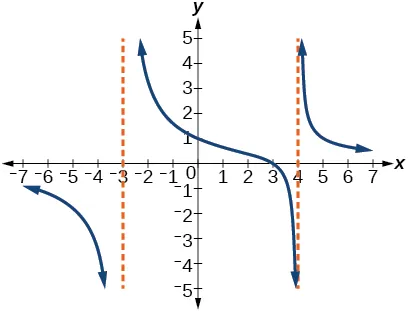58.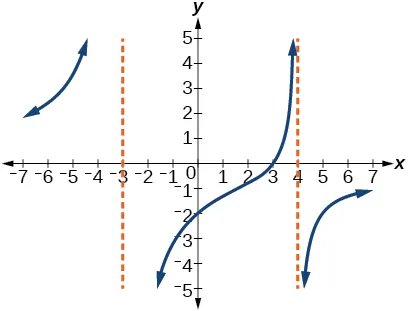59.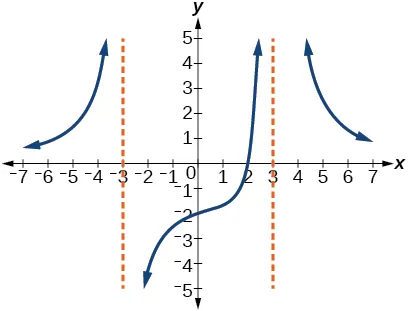60.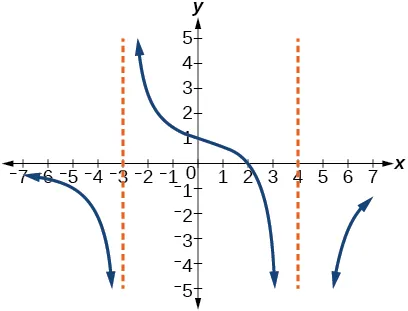61.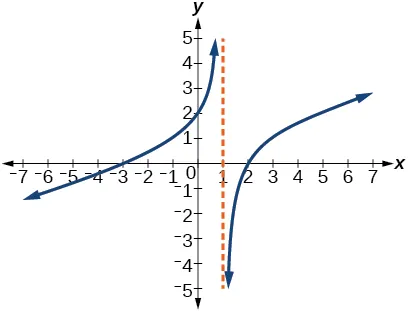62.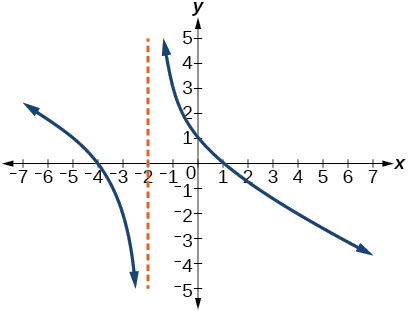63.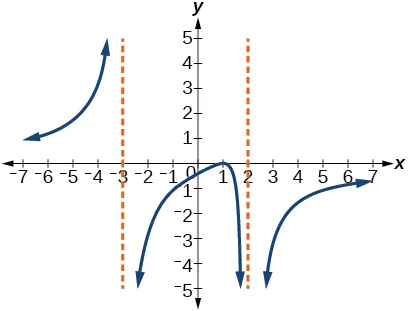64.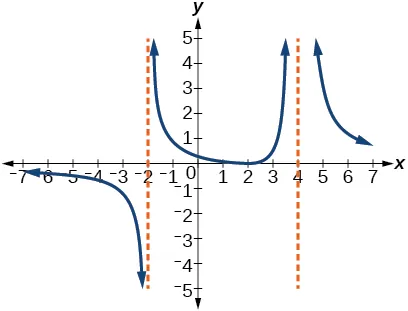#### Numeric

For the following exercises, make tables to show the behavior of the function near the vertical asymptote and reflecting the horizontal asymptote

65.

$f(x)= 1 x−2 f(x)= 1 x−2$

66.

$f(x)= x x−3 f(x)= x x−3$

67.

$f(x)= 2x x+4 f(x)= 2x x+4$

68.

$f(x)= 2x (x−3) 2 f(x)= 2x (x−3) 2$

69.

$f(x)= x 2 x 2 +2x+1 f(x)= x 2 x 2 +2x+1$

#### Technology

For the following exercises, use a calculator to graph $f( x ). f( x ).$ Use the graph to solve $f( x )>0. f( x )>0.$

70.

$f(x)= 2 x+1 f(x)= 2 x+1$

71.

$f(x)= 4 2x−3 f(x)= 4 2x−3$

72.

$f(x)= 2 ( x−1 )( x+2 ) f(x)= 2 ( x−1 )( x+2 )$

73.

$f(x)= x+2 ( x−1 )( x−4 ) f(x)= x+2 ( x−1 )( x−4 )$

74.

$f(x)= (x+3) 2 ( x−1 ) 2 ( x+1 ) f(x)= (x+3) 2 ( x−1 ) 2 ( x+1 )$

#### Extensions

For the following exercises, identify the removable discontinuity.

75.

$f(x)= x 2 −4 x−2 f(x)= x 2 −4 x−2$

76.

$f(x)= x 3 +1 x+1 f(x)= x 3 +1 x+1$

77.

$f(x)= x 2 +x−6 x−2 f(x)= x 2 +x−6 x−2$

78.

$f(x)= 2 x 2 +5x−3 x+3 f(x)= 2 x 2 +5x−3 x+3$

79.

$f(x)= x 3 + x 2 x+1 f(x)= x 3 + x 2 x+1$

#### Real-World Applications

For the following exercises, express a rational function that describes the situation.

80.

In the refugee camp hospital, a large mixing tank currently contains 200 gallons of water, into which 10 pounds of sugar have been mixed. A tap will open, pouring 10 gallons of water per minute into the tank at the same time sugar is poured into the tank at a rate of 3 pounds per minute. Find the concentration (pounds per gallon) of sugar in the tank after $t t$ minutes.

81.

In the refugee camp hospital, a large mixing tank currently contains 300 gallons of water, into which 8 pounds of sugar have been mixed. A tap will open, pouring 20 gallons of water per minute into the tank at the same time sugar is poured into the tank at a rate of 2 pounds per minute. Find the concentration (pounds per gallon) of sugar in the tank after $t t$ minutes.

For the following exercises, use the given rational function to answer the question.

82.

The concentration $C C$ of a drug in a patient’s bloodstream $t t$ hours after injection is given by $C(t)= 2t 3+ t 2 . C(t)= 2t 3+ t 2 .$ What happens to the concentration of the drug as $t t$ increases?

83.

The concentration $C C$ of a drug in a patient’s bloodstream $t t$ hours after injection is given by $C(t)= 100t 2 t 2 +75 . C(t)= 100t 2 t 2 +75 .$ Use a calculator to approximate the time when the concentration is highest.

For the following exercises, construct a rational function that will help solve the problem. Then, use a calculator to answer the question.

84.

An open box with a square base is to have a volume of 108 cubic inches. Find the dimensions of the box that will have minimum surface area. Let $x x$ = length of the side of the base.

85.

A rectangular box with a square base is to have a volume of 20 cubic feet. The material for the base costs 30 cents/ square foot. The material for the sides costs 10 cents/square foot. The material for the top costs 20 cents/square foot. Determine the dimensions that will yield minimum cost. Let $x x$ = length of the side of the base.

86.

A right circular cylinder has volume of 100 cubic inches. Find the radius and height that will yield minimum surface area. Let $x x$ = radius.

87.

A right circular cylinder with no top has a volume of 50 cubic meters. Find the radius that will yield minimum surface area. Let $x x$ = radius.

88.

A right circular cylinder is to have a volume of 40 cubic inches. It costs 4 cents/square inch to construct the top and bottom and 1 cent/square inch to construct the rest of the cylinder. Find the radius to yield minimum cost. Let $x x$ = radius.